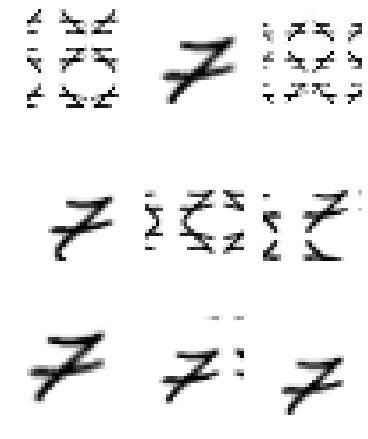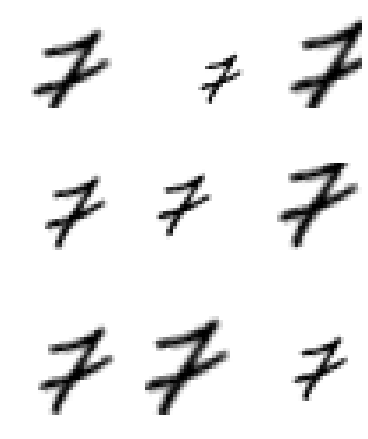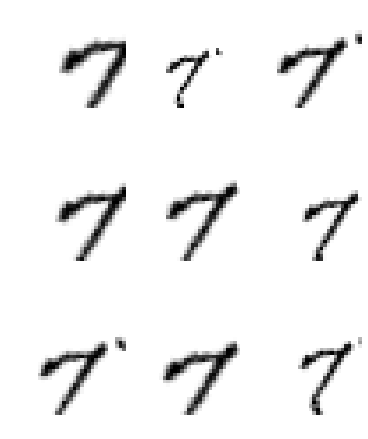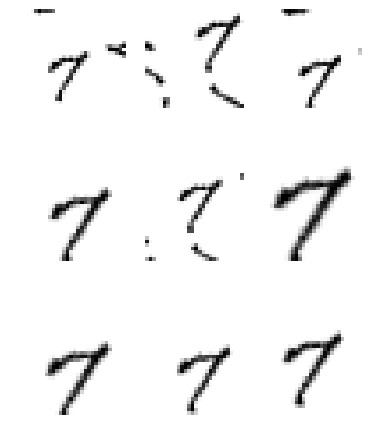# [Solved] Issues (possible Bug) with rand_zoom from the vision transform library

Dear all and team from fastai, recently I joined this wonderful course and this week I started working in a little project using the MNIST data set. While I was trying to do some transformations I think I found a bug (maybe) in the `rand_zoom` method. So before open an issue in github I prefer to ask here first.

This is the minimum code required to reproduce the possible bug.

Starter code (by the way I’m working on colab with the latest fastai version 1.0.52)

``````from fastai.vision import *
path = untar_data(URLs.MNIST)

im_list    = ImageList.from_folder(path, convert_mode='L')
split_data = im_list.split_by_folder(train = 'training', valid = 'testing')
label_data = split_data.label_from_folder().transform(tfms)
data       = label_data.databunch(bs=128).normalize()

``````

and here is where the possible bug happens

``````def get_ex(): return data.train_ds

tfm = rand_zoom(scale=(0.3,1.3))
_, axs = plt.subplots(3,3,figsize=(6,8))
for ax in axs.flatten():
img = get_ex().apply_tfms(tfm)
img.show(ax=ax)
``````I don’t know if this is the intended behavior for the `rand_zoom` transformation but I was expecting a behavior more similar to the cat pictures depicted in the documentation

To be more specific, what I want to achieve is an effect similar to the one provided by these PyTorch transformations

``````transforms.Compose([transforms.RandomAffine(0,
translate=(0.15,0.15), shear=10, scale=(0.3,1.5)),
transforms.RandomRotation(10)
])
``````

because I want to include the following kind of images for my data augmentation

I try to do the equivalent thing with the `fastai` library and I thought this code

``````tfms = ([*rand_pad(padding=3, size=28, mode='zeros'),
rotate(degrees=10),
rand_zoom(scale=(0.6,1.3))], [])
``````

will do the trick, but I found the aforementioned unexpected behavior. Is `rand_zoom` working right? and If `rand_zoom` is working properly, someone knows how I can scale the images in a similar fashion of `scale=(0.3,1.5)` transformation from the torch library

Thanks

You should pass `padding_mode='zeros'` to your call to `transform`, it’s ignored in the `rand_pad` because I’m not sure you’re supposed to pass it there.

1 Like

Thanks @sgugger, your advise solved my problem.

I use `mode = 'zeros'` inside `rand_pad` because I was following the example given in the notebook for the lesson 7 of the course deep learning for coders. I assumed this line

``````rand_pad(padding=3, size=28, mode='zeros')
``````

works like a rigid translation padded with zeros.

Anyway, below I give a full working example for anyone who wants augment his data by shifting, scaling and rotating their images but keeping the same aspect ratio and the width and height for their images

``````tfms = ([*rand_pad(padding=3, size=28, mode='zeros'), rotate(degrees=15), rand_zoom(scale=(0.5,1.3))], [])

im_list    = ImageList.from_folder(path, convert_mode='L')
split_data = im_list.split_by_folder(train = 'training', valid = 'testing')
label_data = split_data.label_from_folder().transform(tfms,  padding_mode='zeros')
data       = label_data.databunch(bs=128).normalize()

def get_ex(): return data.train_ds

_, axs = plt.subplots(3,3,figsize=(6,8))
for ax in axs.flatten():
img = get_ex()
img.show(ax=ax)
``````Important Note: The `mode='zeros'` should be keep inside `rand_pad` because otherwise little reflections start to appearAccording to the documentation of `rand_pad`, `mode='reflection'` is the default mode.

All the above maybe explain the behavior I reported in in my first post, if I use

``````tfms = ([*rand_pad(padding=3, size=28, mode='zeros'), rotate(degrees=15), rand_zoom(scale=(0.5,1.3))], [])
``````

and change this part

``````.transforrm(tfms, padding_mode='reflections')
``````I get something more similar to the image of my first post.

So I conclude that there are two levels in which the padding must be specified to avoid weird results: It must be specified for a kind of “internal” padding for each transformation in which a padding option is available (like in `rand_pad`) and it have to be set for the `fastai.vision.transform` (a kind of general padding) using the key word `padding_mode`

thanks